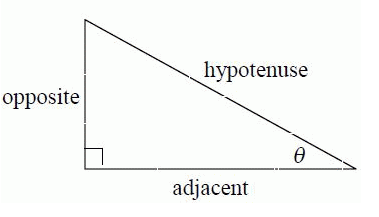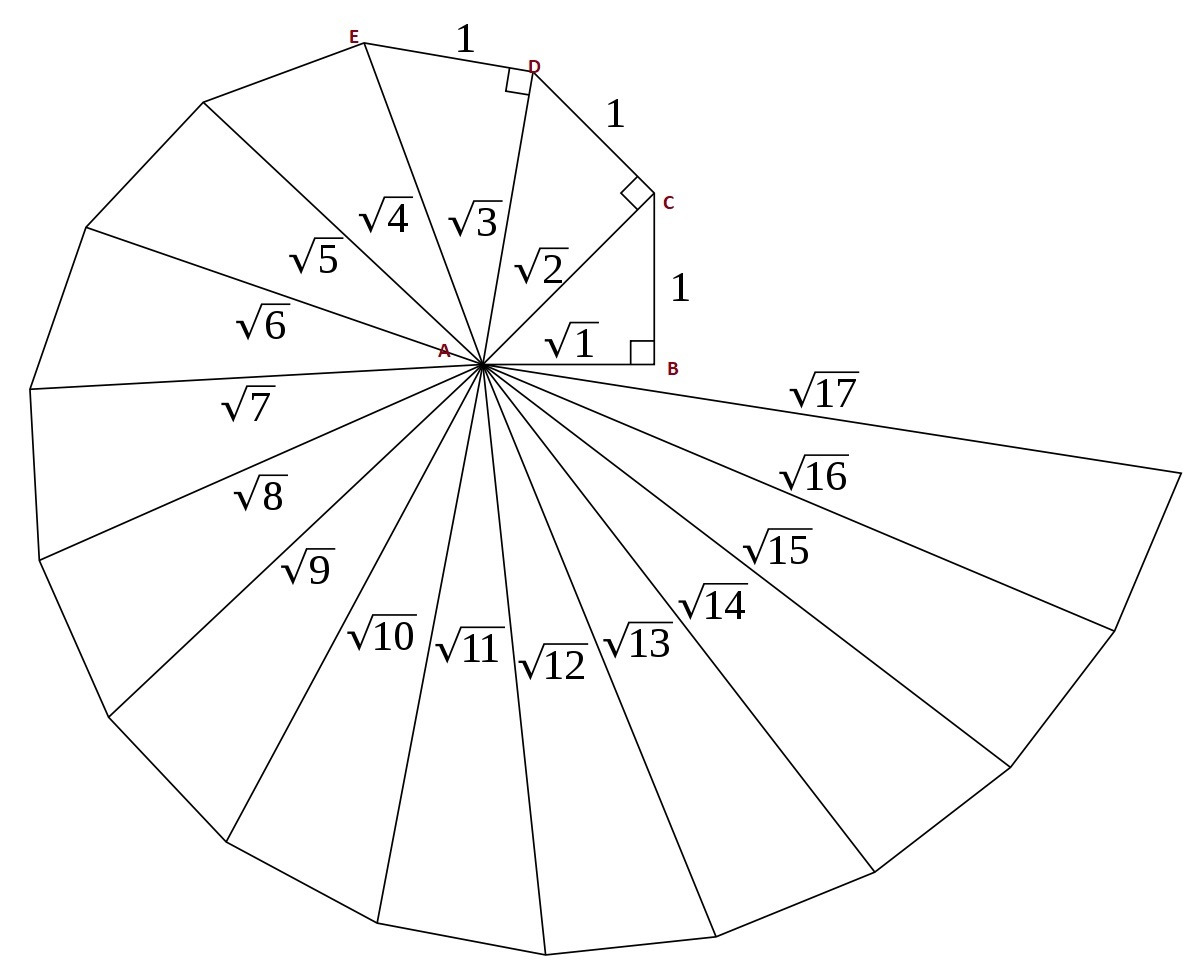# Explain the construction of the square root spiral?

Pythagoras theorem  :

Pythagoras theorem is a fundamental relationship among the three sides of a right triangle.

It states that the area of the square whose side is the hypotenuse is equal to the sum of the areas of the squares on the other two sides. $(h^2= a^2+ b^2)$$h^2= a^2+ b^2$

Where h is the hypotenuse and a, b are the other two sides of the right-angled triangle.

Construction of a Square root spiral:

1. Draw a line segment AB of length 1 unit by taking 1 cm as a unit.

2. Construct a line BX perpendicular to the line segment AB, by using a compass.

3. From B, draw an arc of 1 unit, which cuts BX at C.

4. Join AC.

5. Taking AC as base, draw a perpendicular CY to AC, by using the compass.

6. From C, draw an arc of 1 unit, which cuts CY at D.

8. Taking AD as a base, draw a perpendicular DZ to AD, by using the compass.

9. From D, draw an arc of 1 unit, which cuts DZ at E.

10. Join AE.

11. Keep repeating the above process for a sufficient number of times. Then, the figure so obtained is called a square root spiral.Updated on: 10-Oct-2022

718 Views